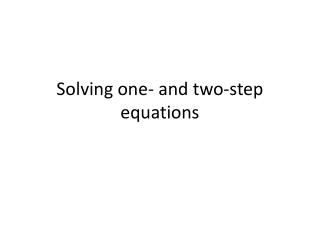# Solving one- and two-step equations - PowerPoint PPT PresentationDownload PresentationSolving one- and two-step equations

Solving one- and two-step equationsDownload Presentation## Solving one- and two-step equations

- - - - - - - - - - - - - - - - - - - - - - - - - - - E N D - - - - - - - - - - - - - - - - - - - - - - - - - - -
##### Presentation Transcript

1. Solving one- and two-step equations

2. Solving One-Step Equations using Additive Inverse Steps Equations X + 4 = 9 -4 -4 x = 5 x – 7 = 5 +7 +7 x = 12 • Given the equation x + 4 = 9 use the inverse of adding 4 to both sides of the equation to isolate the x. (In other words, subtract 4 from each side to get x by itself.) • Given the equation x – 7 = 5 use the inverse of subtracting 7 to both sides. (In other words, add 7 to both sides)

3. Solving One-Step Equations using the Multiplicative Inverse Steps Equations 3x = 9 3x/3 = 9/3 x = 3 x /7 = 5 x/7(7) = 5(7) x = 35 • Given the equation 3x +4 = 9 use the inverse of multiplying 3 to both sides of the equation to isolate the x. (In other words, divide 3 from each side to get x by itself.) • Given the equation x /7 = 5 use the inverse of dividing by 7 to both sides. (In other words, multipy both sides by 7)

4. Solving Two-Step Equations Steps Equations 7x + 5 = 26 -5 -5Step 1 7 x = 21 7x/7 = 21/7 Step 2 x = 3 Given the equation 7x + 5 = 26 • use the inverse of adding 5 to both sides of the equation to isolate the x. • Use the inverse of multiplying by 7 on both sides.

5. Solving Two-Step Equations Steps Equations x/12 - 5 = -1 +5 +5Step 1 x/12 = 4 x/12(12) = 4(12) Step 2 x = 48 Given the equation x/12- 5 = -1 • use the inverse of subtracting 5 to both sides of the equation to isolate the x. • Use the inverse of dividing by 12 on both sides.

6. Solving Equations with the variable on both sides Steps Equation 4x + 10 = 2x +20 -2x -2xStep 1 2x + 10 = 20 -10 -10 Step 2 2x = 10 2x/2 = 10/2 Step 3 X = 5 Given the equation 4x + 10 = 2x + 20 • Subtract 2x from both sides to get the terms with x on one side of the equation. • Subtract 10 from both sides to get numerical terms on the other side. • Divide both sides by 2.

7. Checking your solution Steps Equation X + 4 = 9 -4 -4 x = 5 Check: X + 4 = 9 5 + 4 = 9 • You can check your solution by substituting the value back into your original equation.

8. Checking your solution Steps Equation 7x + 5 = 26 -5 -5Step 1 7 x = 21 7x/7 = 21/7 Step 2 x = 3 Check: 7x + 5 = 26 7(3) + 5 = 26 • You can check your solution by substituting the value back into your original equation.

9. Checking your solution Steps Equation 4x + 10 = 2x +20 -2x -2xStep 1 2x + 10 = 20 -10 -10 Step 2 2x = 10 2x/2 = 10/2 Step 3 X = 5 Check: 4x + 10 = 2x +20 4(5) + 10 = 2(5) + 20 20 + 10 = 10 + 20 30 = 30 • You can check your solution by substituting the value back into your original equation.

10. Ticket out the Door What properties of addition and multiplication can I use to solve one and two step?